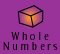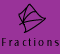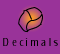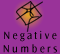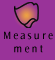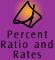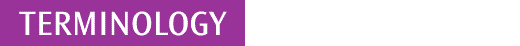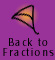Terminology used when working with fractions

denominator:

The denominator is the number on the bottom of a fraction; for example, 4 is the denominator in 3/4. Compare with numerator.

dividend:

The dividend is the number to be divided in a division problem. In the example 3/2 ÷ 4, 3/2 is the dividend.

divisor:

The divisor is the number by which another number is to be divided in a division problem. In the example 3/2 ÷ 4, 4 is the divisor.

fraction:

Fractions are rational numbers whose value can be expressed as a ratio of any 2 whole numbers; say a and b,. (where b cannot be equal to zero, because we cannot divide by zero).

improper fractions: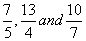are examples of improper fractions because in each case the value of the numerator is greater than the value of the denominator. These fractions have a value greater than one, yet have not been written to show the integer part. Compare with proper fractions and mixed numbers.

We usually call fractions like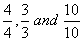improper fractions because they can also be represented by the whole number 1.

inverse:

The inverse of the fraction a/b is the fraction b/a. So, for example, the inverse of 2/5 is 5/2 and the inverse of one and a half (3/2) is 2/3. Multiplying a number by its inverse gives 1. Zero does not have an inverse.

A more correct term for inverse is multiplicative inverse. See also reciprocal.

mixed numbers:

Because improper fractions represent more parts than the whole they can also be expressed as mixed numbers, that is a whole number and a fraction.

We can see below that the improper fractions,can be represented as mixed numbers.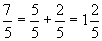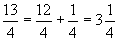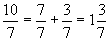If you get confused about what a mixed number represents, think about how we say its value in English.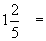one AND two fifths

numerator:

The numerator is the number on top of a fraction; for example, 3 is the numerator in 3/4. Compare with denominator.

proper fractions: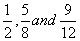are examples of proper fractions because in each case the value of the numerator is less than the value of the denominator. These fractions have a value between 0 and 1. Compare with improper fractions.

quotient:

The quotient is the result of division. In the example 1 ÷ 1/3 = 3, 3 is the quotient.

rational number:

A rational number is a number whose value can be expressed as the ratio of any 2 integers; say a and b,. (where b cannot be equal to zero, because we cannot divide by zero). In this material, we use the term fraction to mean rational number.

Further information: A number such asis not a fraction or a rational number according to our definition. This is because square root 3 is not an integer and cannot be expressed as a ratio of two integers. However,is written in fractional form, and the algorithms for operations on fractions can be applied to it.

reciprocal:

The reciprocal of a fraction a/b is the fraction b/a. So, for example, the reciprocal of 2/5 is 5/2 and the reciprocal of one and a half (3/2) is 2/3. Multiplying a number by its reciprocal gives 1. Zero does not have a reciprocal.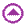A model example for mixed numbers and improper fractions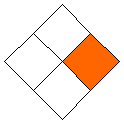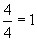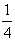Each square has been divided into 4 equal parts to make up a complete square. So we can say 4 parts = 1 whole. If we look at the shaded areas, we have 2 complete squares and one quarter of a square.

What is the improper fraction which describes the model?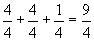What is a mixed number which describes this model?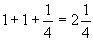You can see that realising that, four quarters equals one, is crucial to us being able to convert improper fractions to mixed numbers and visa versa.